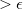# Towards Practical Conditional Risk Minimization

28 Jan 2018

This is the sixth article for the website.

After being inspired by Shagun Sodhani and Philip Guo, I have decided to augment my understanding of my current interests[Computer Vision and Deep Learning] by explaining difficult concepts in easier words.

Disclaimer: This should not be considered impervious to mistakes. I regard myself as just an inquisitive learner and a beginner who wants to learn and help fellow newcomers to learn.

I will be discussing about this paper,Towards Practical Conditional Risk Minimization by Alexander Zimin and Christopher Lampert. Reading research papers is not a trivial task and requires effort in itself. I follow this methodology to read any convoluted research paper.

This paper is based on solving the problem of Conditional Risk Minimization. Mostly, the data we are working on in Machine Learning is an i.i.d data[independent and identically distributed data]. What is this data? Identical and Independent are terms used in Probability. A common example of an identical and independent data can be a throw of a coin:

1. Independent: All throws of coin are independent. No memory is stored about the previous throw.
2. Identical: The resulting distribution is identical which means that we know about the final result: [P(Head) = 1/2, P(Tail) = 1/2].

Coming back to the point from this brief digression, Conditional risk minimization is trying to solve the problem when the data is stochastic. Given a loss function and a set of hypotheses, it aims to minimize the risk of prediction at the next step of a sequentially arriving dependent data. In this case, there can be a dependency[non independent] between the previous and later inputs of the data. What is stochastic data? In probability, stochastic data can be defined as a randomly distributed data with finite constraints. eg. Consider a flag: At any instance of time, it is almost impossible to predict where a point on the flag will be in the next 10 seconds of time. But, we can be sure that its coordinates will be restricted by the boundaries of the flag.

Before moving further, we have to answer a fundamental question, which is: What problem is the entire field trying to solve?. Answering this question is of utmost importance, without which we will not be able to decide whether we need to read this paper. This is my take on this question:

The main problem as covered earlier is to try to work on stochastic data, where there is a dependency between the previous and the next input. For every new input, we would have to predict a new hypothesis which minimizes the risk. Any algorithm which helps to minimize a risk dynamically to 0 is known as limit learner algorithm. The stochastic process we are working on is known as learnable. To learn more about this definitions, look into the previous work by the same author. Also, in the i.i.d learning, we could use Marginal distribution to compute the loss. But this won’t be able to generate information about the long-term behaviour of the data and not short term behaviour of it.(This is because there is a dependency between the inputs).

The next important question we should try to answer is: What exactly are the researchers trying to answer with their research? So, our purpose is to find an algorithm which minimizes the risk for stochastic data. But, the authors have identified the difficulty in finding such an algoirthm. So, they have devised a new metric to calculate such an algorithm:-learning: In epsilon learning, we don’t aim to minimize the risk to 0 but make sure that it is >(threshold).Another metric that the authors are going to use is Discrepancy It is used to compare 2 conditional distributions.

Using the above 2 statistical measures, the authors have devised a new algorithm: MACRO. MACRO computes whether 2 distributions are similar,i.e using discrepancy as a measure and computes a same hypothesis for both of them. Even here, we useas threshold and calculate a new hypothesis if and only if discrepancy. A good algorithm should then be updated only a few times and newer subroutines should be formed rarely.

For MACRO, we can choose several subroutines:

1. Empirical risk minimization: It stores all the points it has been updated with. Then, it outputs a hypothesis that minimizes the output loss over the training set.

2. Online learning The above method is computationally expensive as we are storing all the points it has been updated with. In the case of Online learning, we have to update a suitable predictor at each step.

Conclusion: Summing it all up, we can see the importance of Conditional Risk Minimization for learning stochastic data. We have also seen MACRO which is a suitable algorithm for predicting a suitable algorithm. The future work will now be aimed in finding an optimal value for.

I have tried to cover the paper within my understanding. I have skipped some part like the Mathematics involved in computing the algorithm. I hope to cover it up soon. I would love to hear feedback from you in the comments section!!